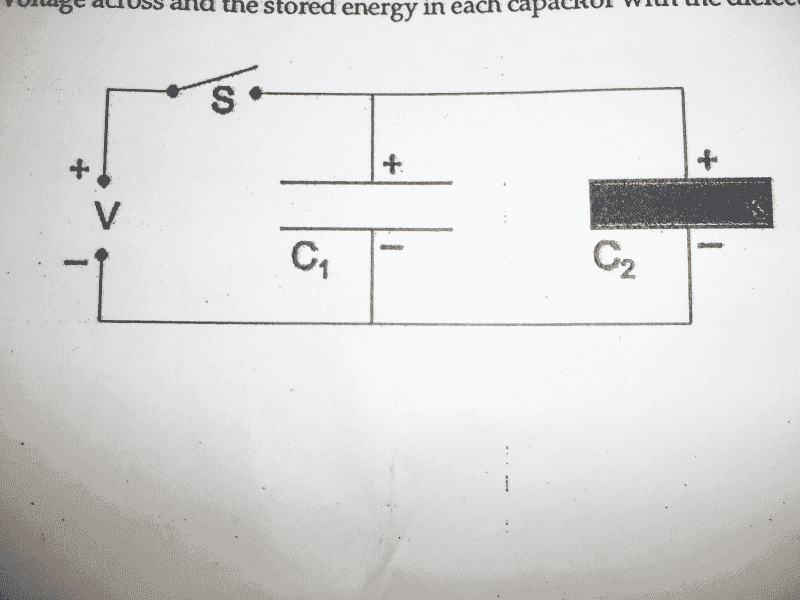# Stored Energy in two Parallel Plate Capacitors

• sweetdion
In summary, two-parallel-plate capacitors with identical plate area and separation are connected to a constant voltage power supply and allowed to fully charge before the switch is opened, disconnecting the power supply. After the switch is opened, the stored energy in each capacitor can be found using the equations U1=CV^2/2 and U2=KCV^2/2. When the dielectric is removed from one of the capacitors, the voltage across and stored energy in each capacitor changes, with the final voltage being (1+k)V and the final energy in each capacitor given by U1=C1((1+k)V)^2/2 and U2=C2((1+k)V)^2/2.

## Homework Statement

The two-parallel-plate capacitors shown, C1=C and C2=KC, have identical plate area and plate separation. The switch S is closed, connecting the capacitors to a constant voltage power supply providing a potential difference of V. The capacitors are allowed to fully charge before the switch is opened again, disconnecting the power supply.
a)After the switch is opened, what is the stored energy in each capacitor?
b) The dielectric is now pulled from the gap of C2 by an external force. What is the voltage across and the stored energy in each capacitor with the dielectric removed?U=CV^2/2
V=Q/C

## The Attempt at a Solution

a) U1=CV^2/2
U2=KCV^2/2
b) When the dielectric is removed from the capacitor, the charge Q on the capacitor remains. Since U = Q2/2C and the capacitance is initially greater by a factor equal to the dielectric constant, C = KC0, removing the dielectric lowers C and increases U. But how do I calculate how much U is increased, and the voltage?

Charge on C1 = Q1 = CV.
Charge on C2 = Q2 = kCV
When the dielectric is removed, the charge Q2 in C2 remains as it is, but voltage changes.So V' = Q2/C or Q2 = C*V'
Since the capacitors are connected in parallel, the common potential is
Vf = (Q1+Q2)/2C. = (CV+kCV)/(2C) = (1+k)V.
Now find the energy in each capacitor.

Thankz rl.bhat.

I get U1=C1((1+k)V)^2/2
U2=C2((1+k)V)^2/2

Right?

Last edited: